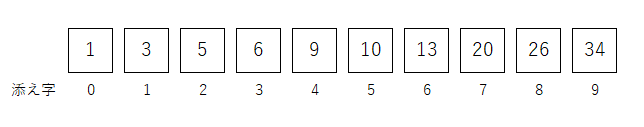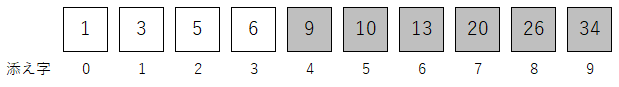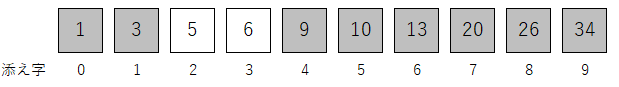# [RUBY] Use a binary search to see if there are any values in the array

It's a story that many people have already written, I wish I could deepen my understanding by writing, so I will write it.

## problem

Let's solve the following problem using binary search.

There is an array ʻarray = [1, 3, 5, 6, 9, 10, 13, 20, 26, 34]`, Write code to find out if any value exists in this array. If any value does not exist in the array, "The value does not exist in the array" is displayed and If it exists, the number in the array is displayed.

``````#Output example 1
Please enter the number you want to search
5
5 is second in the array

#Output example 2
Please enter the number you want to search
8
8 does not exist in the array
``````

## What is a binary search in the first place?

When searching for sorted lists or data in arrays (assuming they do not have the same value) Look at the value in the center and use the magnitude relationship with the value you want to search, Determine if the value you want to search for is to the right or left of the center value, A method of searching while making sure that it does not exist on one side. Since the choices are halved in one process, improvement in processing speed can be expected. Also called binary search or binary search.

## I will write roughly what it is like

The value you want to search for is `target`, The leftmost subscript is `left`, The rightmost subscript is `right`, The subscript in the middle is assigned to `center`.

By the way, this time I will search with target = 5.

The diagram of the array is as follows.Let's actually try it.

In the first search left = 0 right = 9 And the subscript with the median value is center = (left + right) / 2, that is, center = 4. (Actually, 9 divided by 2 is 4.5, but in the case of Ruby, integer / integer = integer (rounded down to the nearest whole number), so this is okay) Now that you know the subscript with the median value, compare it with the value you want to search. array[center] = 9 target = 5 So array [center]> target. Now you know the range to search next. It looks like the figure below. Gray is out of search.As you can see in the figure, the right changes. Since it will be changed to the left side of the center right = center - 1

Of course, the center also changes. The method of finding is the same as the first time. center = (left + right) / 2 By the way, the second calculation gives center = 1.

Then, like the first time, compare the center value with the value you want to search. array[center] = 3 target = 5 So array [center] <target.

And you can see the next search range.This time left changes. Because it will be one right of the center left = center + 1 The center also changes. The method of finding is the same as before. center = (left + right) / 2 By the way, the third time is calculated as center = 2.

Then compare the median value with the value you want to search as before. array[center] = 5 target = 5 array [center] == target and the search ends.

I will rewrite it while organizing what I wrote roughly above.

``````def binary_search(array, right, target)
left = 0
while left <= right
center = (left + right) / 2
if array[center] == target
return center
elsif array[center] > target
right = center - 1
else
left = center + 1
end
end
return -1
end

array = [1, 3, 5, 6, 9, 10, 13, 20, 26, 34]  #16th line

puts "Please enter the number you want to search"  #18th line
target = gets.to_i
elements_num = array.count - 1

result = binary_search(array, elements_num, target)  #Line 22

if result == -1  #24th line
puts "#{target}Does not exist in the array"
else
puts "#{target}Is an array#{result}Exists second"
end
``````

### Supplement

There is a description about binary search in the binary_search method.

Set to repeat processing with while. The condition for the repetition to be valid is until the leftmost subscript (left) of the array becomes the same as the rightmost subscript (right) of the array (when it exceeds, the processing in while is not performed). Let left <= right.

If array [center] == target, I am trying to return the value of center and get out of the process in the method with return.

Line 20 elements_num = array.count --1 gets the rightmost subscript of the array. Maybe length is fine instead of count.

## If it's an unsorted array

You can sort by `array name.sort`.

## end

There is a way to compare using each method, but the more the contents of the array, the more convenient the binary search is. By the way, I wonder if a certain app that was popular with friends used this mechanism in the past.

It's been a long time. Thank you for staying with us until the end.